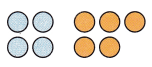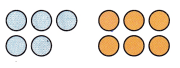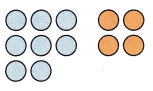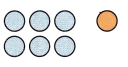All the solutions provided in McGraw Hill Math Grade 1 Answer Key PDF Chapter 2 Lesson 2 Addition Facts Through 12 as per the latest syllabus guidelines.

Write the sum.

Question 1.Question 2.4 + 5 = ____
Explanation:
There are 4 blue circles and 5 brown circles in the above figure
By adding 4 and 5 we get 9.
4 + 5 = 9

Question 3.5 + 6 = ___
Explanation:
There are 5 blue circles and 6 brown circles in the above figure
By adding 5 and 6 we get 11.

Question 4.8 + 4 = ___
Explanation:
There are 8 blue circles and 4 brown circles in the above figure
By adding 8 and 4 we get 12.

Question 5.6 + 1 = ___